### Author Archive

[latexpage]

Circle graphs (also called pie charts) let you see the relative amounts of different categories. For example, if you run a local grocery store and want to see where your sales are coming from (perhaps because you are considering whether to re-allocate floor space), you might look at a chart like the following: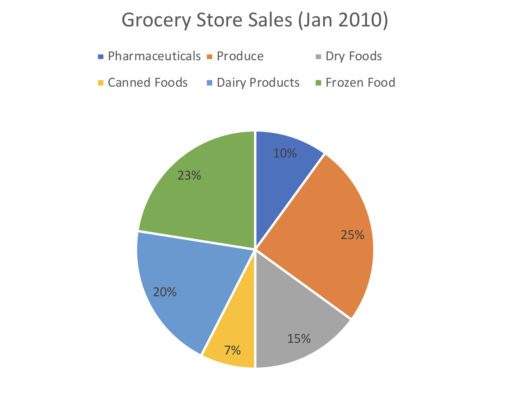and conclude that as most of your sales come from produce, you may want to allocate more space to new kinds of produce.

Now, when reading a circle graph, the percentages of the different sections will generally be labelled as in the above. So circle graph tells you that in January of 2010, 23% of all sales were from frozen foods, 10% of all sales were from pharmaceuticals, and so on.

Now, if you are given the actual value (as opposed to the proportion) of any category, you can find the value for each category. So, for example:

Example 1

Suppose that in January of 2010, the grocery store sold \$23,000 worth of frozen foods. How many dollars worth of canned foods did they sell? We can also use circle graphs to determine various trends. For example, by comparing the following charts: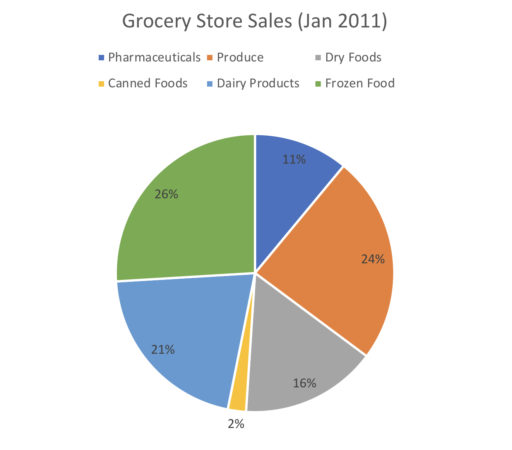we can conclude that the proportion of revenue from canned foods drastically shrunk from January 2010 to 2011. Example 2 From January 2010 to January 2011, which category grew the most as a proportion of total sales? Now, if we know the actual value of some category in 2010 and the actual value of some category in 2011, we can calculate the value of each category and 2010 and 2011. Thus, we can calculate the absolute increase in revenue for any particular category as follows: Example 3 Suppose in January 2010, the total amount of goods sold was \$200,000. In January 2011, the amount of canned foods sold was \$6,000. Find the absolute change from 2010 to 2011 in the amount of dairy products sold. Practice Problems 1. Suppose produce sales were, in absolute terms, \$10,000 greater than pharmaceutical sales in January 2010. What was the total amount of sales for all goods?2. Overall sales in January 2010 were \$100,000. How much more revenue was generated by dry foods as compared to canned foods?3. Suppose dry foods in January 2010 were twice as large, in absolute terms, as pharmaceutical sales in January 2011. What is the ratio of overall sales from January 2010 to January 2011?Comment on this A box plot is so named for its iconic shape: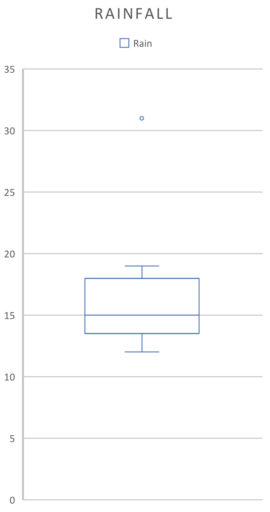(They can also be laid out horizontally). The parts of the graph correspond to: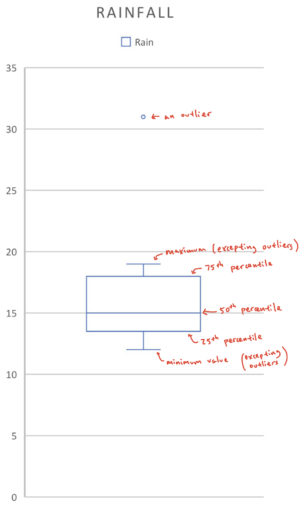Looking at a box plot can give you a quick sense of what the distribution of the data looks like. For example: Example 1 Find the 25th percentile, 50th percentile, 75th percentile, range, and median for the below boxplot: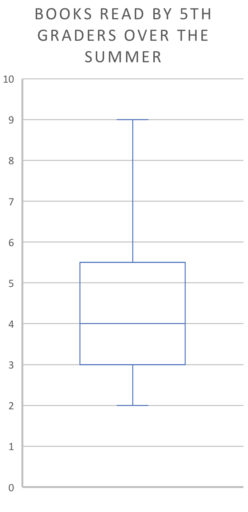Example 2 The following chart represents the books read by the 500 fifth-graders in a school over the summer. Suppose no student read exactly 4 books. How many students read more than 4 books?Example 3 The following chart represents the books read by the 500 fifth-graders in a school over the summer. What is the approximate number of students that have read between 3 and 4 books?Practice Problems 1. The following chart represents the books read by the 100 fifth-graders in a school over the summer. What is the approximate number of students that have read more than 5 books? (We assume that people can only read positive integers of books).2. The following chart represents the books read by the 500 fifth-graders in a school over the summer. Suppose 7 is the 80th percentile of this data. Approximately how many people read between 6 and 7 books? (We assume that people can only read positive integers of books).3. Researchers measured, over 36 months, how often it rained in a month. About how many months had rain from between 13 and 18 days?Comment on this [latexpage] Talk of "data" is ubiquitous -- what does that word mean in the context of the GRE? We will think about data as observations of given variables. Now what does that mean? Well suppose we are trying to help our child increase her earnings from a lemonade stand. Some days, she sells a lot and some days she sells almost nothing. To figure out why, we might start keeping track of how much she sells on a given day. So we would start making a table that looks like: $$\begin{center} \begin{tabular}{ |c|c|c| } \hline Date & Lemonades Sold \\ \hline 7/2/19 & 1 \\ \hline 7/3/19 & 2 \\ \hline 7/4/19 & 5 \\ \hline 7/5/19 & 2 \\ \hline 7/6/19 & 3 \\ \hline \end{tabular} \end{center}$$ Now, each of the rows in the table is an observation. And we have our variables at the top of the columns: the date and the number of lemonades sold. And more generally: variables are just the characteristics that we keep track of, while an observation is some particular occasion when we record the values of our variables. Now, a very simple way to keep track of data is via a frequency distribution. This is a table that records, on the left-hand side, possible values of a given variable, and on the right-hand side, it records how often those values appeared. Applied to the above data, we would get: $$\begin{center} \begin{tabular}{ |c|c| } \hline Lemonades Sold & Number of Days\\ \hline 1 & 1 \\ \hline 2 & 2 \\ \hline 3 & 1 \\ \hline 4 & 0 \\ \hline 5 & 1 \\ \hline \end{tabular} \end{center}$$ This table answers questions like "How often did my child sell three lemonades?" To find the answer, we go to the "Lemonades Sold" column and look for the row with three lemonades sold. In that row, the right hand column (corresponding to the number of days) says one. So there was one day where the child sold three lemonades. And, in addition to a frequency distribution, we can also create a relative frequency distribution which records, on the left-hand side, possible values of a given variable, and on the right hand-side, the percentage of all observations where that value occurred. So, in the above example, we would get: $$\begin{center} \begin{tabular}{ |c|c| } \hline Lemonades Sold & Number of Days\\ \hline 1 & 20\% \\ \hline 2 & 40\% \\ \hline 3 & 20\% \\ \hline 4 & 0\% \\ \hline 5 & 20\% \\ \hline \end{tabular} \end{center}$$ since the total number of days is$1 + 2 + 1 + 0 + 1 = 5$and$\frac{1}{5} =$20% and$\frac{2}{5} =\$ 40% and so on through the table.

Now, to really get a handle on what is driving her lemonade sales, we should probably add some more variables (e.g. daily temperature, day of week) and collect some more observations:

$$\begin{center} \begin{tabular}{ |c|c|c|c| } \hline Date & Lemonades Sold & Day of Week & Temperature (Fahrenheit)\\ \hline 7/2/19 & 1 & Tuesday & 68 \\ \hline 7/3/19 & 2 & Wednesday & 73 \\ \hline 7/4/19 & 5 & Thursday & 75 \\ \hline 7/5/19 & 2 & Friday & 70 \\ \hline 7/6/19 & 3 & Saturday & 71\\ \hline 7/7/19 & 2 & Sunday & 71 \\ \hline 7/8/19 & 5 & Monday & 78\\ \hline 7/9/19 & 4 & Tuesday & 75 \\ \hline 7/10/19 & 3 & Wednesday & 72\\ \hline 7/11/19 & 3 & Thursday & 73\\ \hline \end{tabular} \end{center}$$

Here are some practice problems on the above concepts:

Practice Problems:

1. Using the above data, construct a frequency table that tells you how often a certain number of lemonades was sold:
2. Using the above data, how many lemonades were sold on Tuesdays?
3. Using the above data, how many days had more than 3 lemonades sales?
4. On days where the temperature was at least 73 degrees, how many lemonades did she sell on average?
5. On days where the temperature was less than 73 degrees, how many lemonades did she sell on average?

Comment on this

In the next few posts, we’ll unpack some of the statistical terms that the GRE throws around. These terms can be seen as ways to answer two central questions:

1. What’s Typical?
2. What’s Possible?

In response to the question of "What's Typical?" we can find the median, mean, or mode of the data. These give you a sense of what the average value is, or what the most common value is; in short, they describe what a typical value might be.

And in answering "What's Possible?" we can find the range, quartiles, or percentiles of the data. These give you a sense of the possibilities and how the possibilities are distributed.

In later posts, we will give actual definitions of these concepts. But first, some overarching advice about learning these concepts.

There are four main ways you’ll get tested on these concepts.

1. Given some data, find the value of one of these concepts (e.g. the mean, median, range, etc.).
2. Given some data, alter the data in some way (e.g. by adding 10 to every value), and then find the value of one of these concepts.
3. Given some graph/chart, estimate the value of one of these concepts.
4. Answer some question about some concept's general properties (e.g. that the interquartile range is always less than or equal to the range).

Thus, you want to understand the formal definition well enough to do questions of the first and second types. But you also want to have some intuitive sense for the concepts, so that you can do questions that fall in the third and fourth categories.

Comment on this